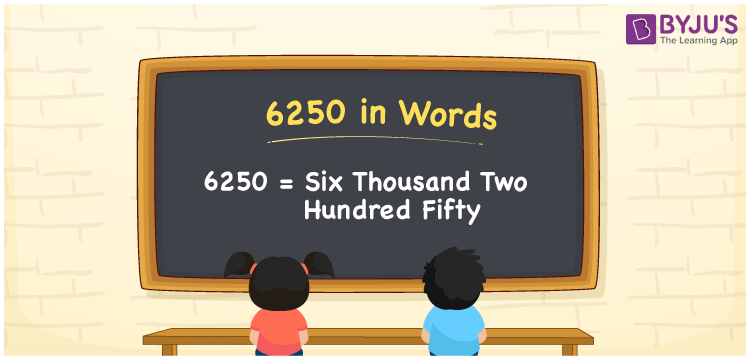# 6250 in Words

6250 in words can be written as Six Thousand Two Hundred Fifty. If you buy a cotton dress for Rs. 6250, then you can say that “I bought a cotton dress for Six Thousand Two Hundred Fifty Rupees”. This article will provide you with a clear idea of how numbers in words can be written using the English language. Therefore, the number 6250 can be read as “Six Thousand Two Hundred Fifty” in words.

 6250 in words Six Thousand Two Hundred Fifty Six Thousand Two Hundred Fifty in Numbers 6250

## 6250 in English Words## How to Write 6250 in Words?

The place value chart of 6250 is shown here in the form of a table. 6250 has four digits and the place value of each can be known from the table here.

 Thousands Hundreds Tens Ones 6 2 5 0

The expanded form of 6250 is provided below:

6 x Thousand + 2 x Hundred + 5 × Ten + 0 × One

= 6 x 1000 + 2 x 100 + 5 x 10

= 6000 + 200 + 50

= 6250

= Six Thousand Two Hundred Fifty

Therefore, 6250 in words is written as Six Thousand Two Hundred Fifty.

6250 is a natural number that precedes 6251 and succeeds 6249.

6250 in words – Six Thousand Two Hundred Fifty

Is 6250 an odd number? – No

Is 6250 an even number? – Yes

Is 6250 a perfect square number? – No

Is 6250 a perfect cube number? – No

Is 6250 a prime number? – No

Is 6250 a composite number? – Yes

## Frequently Asked Questions on 6250 in Words

Q1

### How do you write the number 6250 in words?

6250 in words is Six Thousand Two Hundred Fifty.
Q2

### Find the value of 6300 minus 50.

The value of 6300 minus 50 is 6250. Therefore, 6250 in words is Six Thousand Two Hundred Fifty.
Q3

### Is 6250 a perfect square?

No, 6250 is not a perfect square as it cannot be expressed as the product of two integers which are equal.